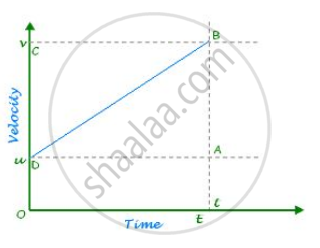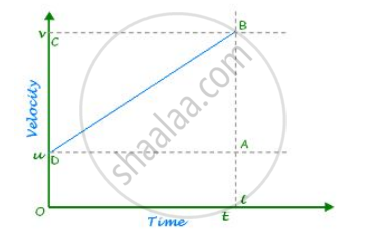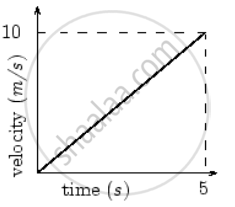# Kinematic Equations for Uniformly Accelerated Motion

#### description

• FIRST METHOD v = vo + at

• SECOND METHOD y = y + v t + at^2

## Kinematic Equations of Motion

If an object starts with velocity ”u” and after some time “t” its velocity changes to v, if the uniform acceleration is a and distance travelled in time (t) is s, then we obtain the following kinematic equations of uniformly accelerated motion.

First Equation Of MotionAs shown in the graph above,
Let an object is moving with uniform acceleration
u = initial velocity of the object
v = final velocity of object
a = uniform acceleration
Let object reach point B after time (t) Now, from the graph

Slope= Acceleration(a)=change in velocity/time
Change in velocity = AB =bar v-bar u
a=(bar v- bar u)/t
Solving this we get the first equation of motion:
bar v=bar u+a bar t

Second Equation Of MotionConsider an object starting with an initial velocity u and moving with uniform acceleration a as shown in the graph above. Distance covered by the object at the given time t is given by the area of the trapezium ABDOE. Let in the given time ‘t’, the displacement covered by the moving object ‘s’ is given by the area of a trapezium, ABDOE

Displacement (s) = Area of ABD + Area of ADOE

1/2 × AB× AD+ AE ×OE, AB= dc= at

=1/2 at× t+ ut

Hence, the second equation of motion is:
bar s=bar ut +1/2 bar a t^2

Third Equation Of Motion
Velocity time graph of a uniformly accelerated motionThe velocity-time graph of a uniformly accelerated motion is a straight line graph inclining towards the time axis. If the object has positive constant acceleration, the graph slopes upward. In case the object has negative constant acceleration, the velocity-time graph will slope downward.

If you would like to contribute notes or other learning material, please submit them using the button below.